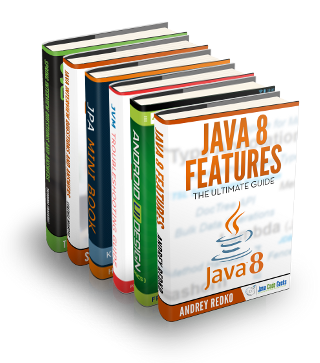Software Development

# INTERSECT – the Underestimated Two-Way IN Predicate

Have you ever wondered how you could express a predicate that “feels” like the following, in SQL:

`WHERE Var1 OR Var2 IN (1, 2, 3)`

/u/CyBerg90 has, on reddit. The idea was to create a predicate that yields true whenever both values `Var1` and `Var2` yield either 1, 2, or 3.

## The canonical solution

The canonical solution would obviously be to write it all out as:

```WHERE Var1 = 1 OR Var1 = 2 OR Var1 = 3
OR    Var2 = 1 OR Var2 = 2 OR Var2 = 3```

A lot of duplication, though.

## Using IN predicates

Most readers would just connect the two `IN` predicates:

```WHERE Var1 IN (1, 2, 3)
OR    Var2 IN (1, 2, 3)```

Or the clever ones might reverse the predicates as such, to form the equivalent:

```WHERE 1 IN (Var1, Var2)
OR    2 IN (Var1, Var2)
OR    3 IN (Var1, Var2)```

## Nicer solution using EXISTS and JOIN

All of the previous solutions require syntax / expression repetition to some extent. While this may not have any significant impact performance-wise, it can definitely explode in terms of expression length. Better solutions (from that perspective) make use of the `EXISTS` predicate, constructing ad-hoc sets that are non-empty when both `Var1` and `Var2` yield either 1, 2, or 3.

Here’s `EXISTS` with `JOIN`

```WHERE EXISTS (
SELECT 1
FROM (VALUES (Var1), (Var2)) t1(v)
JOIN (VALUES (1), (2), (3)) t2(v)
ON t1.v = t2.v
)```

This solution constructs two tables with a single value each, joining them on that value:

```+------+    +------+
| t1.v |    | t2.v |
+------+    +------+
| Var1 |    |    1 |
| Var2 |    |    2 |
+------+    |    3 |
+------+```

Looking at a Venn Diagram, it is easy to see how JOIN will produce only those values from `t1` and `t2` that are present in both sets:

## Nicest solution using EXISTS and INTERSECT

However, people might not think of a set intersection when they read `JOIN`. So why not make use of actual set intersection via `INTERSECT`? The following is the nicest solution in my opinion:

```WHERE EXISTS (
SELECT v
FROM (VALUES (Var1), (Var2)) t1(v)
INTERSECT
SELECT v
FROM (VALUES (1), (2), (3)) t2(v)
)```

Observe, how the length of the SQL statement increases with `O(m + n)` (or simply `O(N)`, where `m, n = number of values in each set`, whereas the original solutions using `IN` increase with `O(m * n)` (or simply `O(N2)`).

#### INTERSECT Support in popular RDBMS

`INTERSECT` is widely supported, both in the SQL standard as well as in any of the following RDBMS that are supported by jOOQ:

• CUBRID
• DB2
• Derby
• H2
• HANA
• HSQLDB
• Informix
• Ingres
• Oracle
• PostgreSQL
• SQLite
• SQL Server
• Sybase ASE
• Sybase SQL Anywhere

In fact, the following databases also support the less commonly used `INTERSECT ALL`, which doesn’t remove duplicate values from resulting bags (see also `UNION` vs. `UNION ALL`)

• CUBRID
• PostgreSQL

Happy intersecting!

 Reference: INTERSECT – the Underestimated Two-Way IN Predicate from our JCG partner Lukas Eder at the JAVA, SQL, AND JOOQ blog.

## Want to know how to develop your skillset to become a Java Rockstar?### Lukas Eder

Lukas is a Java and SQL enthusiast developer. He created the Data Geekery GmbH. He is the creator of jOOQ, a comprehensive SQL library for Java, and he is blogging mostly about these three topics: Java, SQL and jOOQ.
Subscribe
Notify ofThis site uses Akismet to reduce spam. Learn how your comment data is processed.

Inline Feedbacks